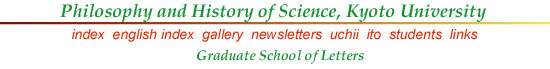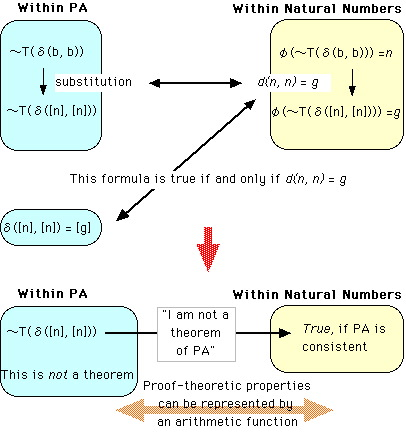Tarski's Theorem

The reason why Goedel's Theorem holds may become clearer if you look at another theorem due to Alfred Tarski, called Tarski's Theorem. Instead of the predicate of theorem T(a) in PA, "a is a theorem", consider another predicate Tr(a), "a is true"; what would happen if this predicate were definable in PA? It is widely recognized that the predicate of truth must satisfy the following condition:

(1) The sentence "p" is true if and only if p.

Namely, by referring to the sentence by a suitable expression (a name, or a description), you would have an equivalence such as this between the attribution of truth to that sentence and that sentence itself. In terms of Goedel number of a sentence A, (1) becomes:

(2) Tr(āė(A)) ü▀ A

Now the question is: Can we define this predicate Tr() in PA? The answer should now be clear to the reader who knows the Halting Problem and Goedel's Theorem. We cannot define, because that would produce a contradiction in PA. For, recall the previous figure:Throughout this figure, replace T() by Tr(). Then, Goedel's unprovable yet true formula becomes:

(3) ü`Tr(ā┬([n], [n]))

and this says "I am not true" (ā┬([n], [n]) signifies the Goedel number of (3) itself); but then, if it is true it is not true, and if it is not true it must be true! Or more formally (according to (2)),

(4) Tr(ā┬([n], [n])) ü▀ ü`Tr(ā┬([n], [n])).

This is a plain contradiction. Thus, if PA is to be consistent, we cannot define Tr() with the property of (1) or (2). This is Tarski's Theorem. As you have already noticed, this has a close connection with the Liar Paradox. Goedel's Theorem may be regarded as a corollary from Tarski's Theorem, because if the truth and the theorem of PA coincides, a contradiction follows immediately; thus there must be a truth which is not a theorem.